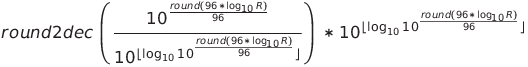EIA Standard Resistor Value Approximation in JavaScript

While working on my bi-directional resistor color code calculator, one of the first things I stumbled upon was how to normalize the user supplied resistance value.
Generally, resistors come in predefined values, or “preferred values”. These values were defined by Electronic Industries Association (EIA) (IEC 60038) and consist of about 6 different series with each series corresponding to particular tolerances:

``````E6    20% tolerance
E12   10% tolerance
E24   5% tolerance
E48   2% tolerance
E96   1% tolerance
E192  0.5, 0.25, 0.1% tolerances
``````

Full table

Such division ensures that substituting an arbitrary value with nearest preferred value wouldn’t exceed the required tolerance. In other words, given a tolerance and an arbitrary resistance value we can calculate the preferred value using the above table.

Fortunately there is much neater way to do this. It is possible to approximate E-series preferred values (which is basically Renard series with different interval steeping) with the following equation (author unknown):Where R is the resistance, round() is round to nearest integer function, and round2decimal() is round to 2 decimal places function.
The equation seemed to work for E48 and E96 series, but gave wrong values for other. The code below is its adaptation for JavaScript and for the rest of the series E12/24/192. The function accepts resistance value in Ohm plus tolerance and produces closest preferred value.